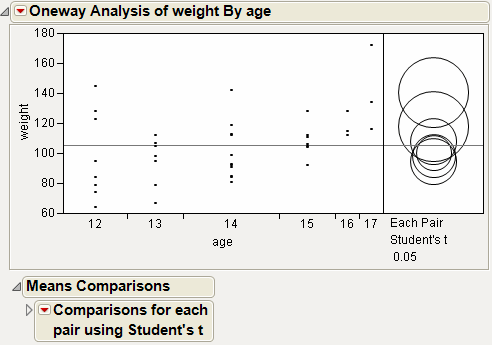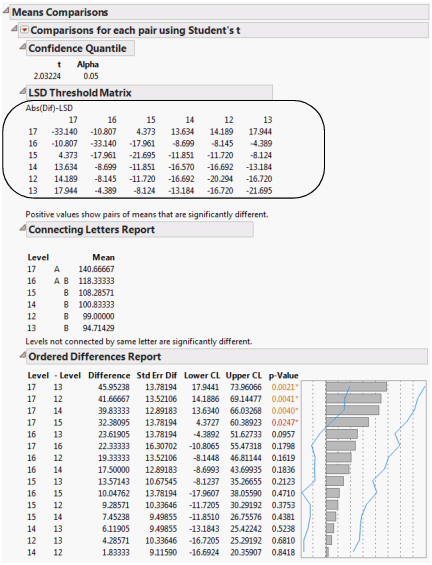Basic Analysis > Oneway Analysis > Additional Examples of the Oneway Platform > Example of the Each Pair, Student’s t Test
Publication date: 05/24/2021

## Example of the Each Pair, Student’s t Test

This example illustrates the use of all possible t tests.

1. Select Help > Sample Data Library and open Big Class.jmp.

2. Select Analyze > Fit Y by X.

3. Select weight and click Y, Response.

4. Select age and click X, Factor.

5. Click OK.

6. Click the red triangle next to Oneway Analysis of weight By age and select Compare Means > Each Pair, Student’s t.

Figure 6.15 Example of Each Pair, Student’s t Comparison CirclesThe means comparison method can be thought of as seeing if the actual difference in the means is greater than the difference that would be significant. This difference is called the LSD (least significant difference). The LSD term is used for Student’s t intervals and in context with intervals for other tests. In the comparison circles graph, the distance between the circles’ centers represent the actual difference. The LSD is what the distance would be if the circles intersected at right angles.

Figure 6.16 Example of Means Comparisons Report for Each Pair, Student’s tIn Figure 6.16, the LSD threshold table shows the difference between the absolute difference in the means and the LSD (least significant difference). If the values are positive, the difference in the two means is larger than the LSD, and the two groups are significantly different.